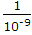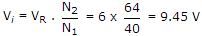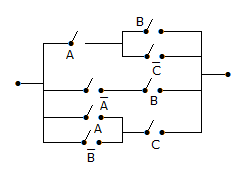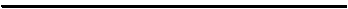# Electronics and Communication Engineering - Digital Electronics

36.

A pulse train with a 1 MHz frequency is counted using a 1024 modulus ripple counter using JK flip flops. The maximum propagation delay for each flip-flop should be

 A. 1 μs B. 0.5 μs C. 0.2 μs D. 0.1 μs

Answer: Option D

Explanation:

Total delay == 1 ms = 10 x delay of one flip flop. Therefore delay for one flip-flop = 0.1 ms.

37.

What will be maximum input that can be converted for a 6 bit dual slope A/D converter uses a reference of -6v and a 1 MHz clock. It uses a fixed count of 40 (101000).

 A. 9 V B. 9.45 C. 10 V D. 8 V

Answer: Option B

Explanation:.

38.

In the given figure shows a logic circuit. The minimum Boolean expression for this circuit isA. A + B B. A + B + C C. AB + C D. AB + AC + BC

Answer: Option B

Explanation:

Output A(B + C) + AB + C(A + B) = AB + AC + AB + AC + BC = B + A + BC = A + B + C.

39.

If number of information bits is 11, the number of parity bits in Hamming code is

 A. 5 B. 4 C. 3 D. 2

Answer: Option B

Explanation:

2p > m + p + 1. If m = 11, p must be 4 to satisfy this equation.

40.

The number of digit 1 present in the binary representation of 3 x 512 + 7 x 64 + 5 x 8 + 3 is

 A. 8 B. 9 C. 10 D. 12

Answer: Option B

Explanation:

3 x 512 + 7 x 64 + 5 x 8 + 3

= (2 + 1) x 29 + (4 + 2 + 1)26 + (4 + 1)23 + (2 + 1)

= 210 + 29 + 28 + 27 + 26 + 25 + 23 + 21 + 1

= 210 + 29 + 28 + 27 + 26 + 25 + 23 + 21 + 209 term of power two

Hence number of '1' is 9.

#### Current Affairs 2021

Interview Questions and Answers